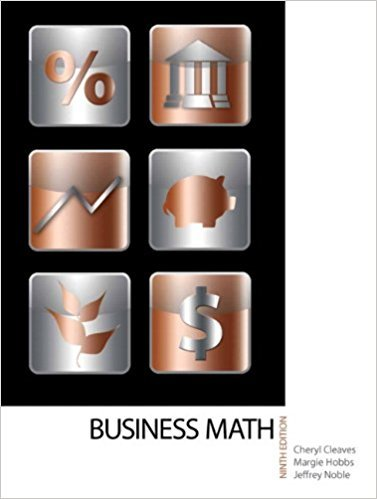×
×

# Solutions for Chapter 21-3: FINANCIAL STATEMENT RATIOS## Full solutions for Business Math, | 9th Edition

ISBN: 9780135108178Solutions for Chapter 21-3: FINANCIAL STATEMENT RATIOS

Solutions for Chapter 21-3
4 5 0 247 Reviews
20
4
##### ISBN: 9780135108178

This textbook survival guide was created for the textbook: Business Math, , edition: 9. Chapter 21-3: FINANCIAL STATEMENT RATIOS includes 8 full step-by-step solutions. This expansive textbook survival guide covers the following chapters and their solutions. Since 8 problems in chapter 21-3: FINANCIAL STATEMENT RATIOS have been answered, more than 17375 students have viewed full step-by-step solutions from this chapter. Business Math, was written by and is associated to the ISBN: 9780135108178.

Key Math Terms and definitions covered in this textbook
• Back substitution.

Upper triangular systems are solved in reverse order Xn to Xl.

• Companion matrix.

Put CI, ... ,Cn in row n and put n - 1 ones just above the main diagonal. Then det(A - AI) = ±(CI + c2A + C3A 2 + .•. + cnA n-l - An).

A sequence of steps (end of Chapter 9) to solve positive definite Ax = b by minimizing !x T Ax - x Tb over growing Krylov subspaces.

• Diagonalization

A = S-1 AS. A = eigenvalue matrix and S = eigenvector matrix of A. A must have n independent eigenvectors to make S invertible. All Ak = SA k S-I.

• Echelon matrix U.

The first nonzero entry (the pivot) in each row comes in a later column than the pivot in the previous row. All zero rows come last.

• Fast Fourier Transform (FFT).

A factorization of the Fourier matrix Fn into e = log2 n matrices Si times a permutation. Each Si needs only nl2 multiplications, so Fnx and Fn-1c can be computed with ne/2 multiplications. Revolutionary.

• Free variable Xi.

Column i has no pivot in elimination. We can give the n - r free variables any values, then Ax = b determines the r pivot variables (if solvable!).

• Jordan form 1 = M- 1 AM.

If A has s independent eigenvectors, its "generalized" eigenvector matrix M gives 1 = diag(lt, ... , 1s). The block his Akh +Nk where Nk has 1 's on diagonall. Each block has one eigenvalue Ak and one eigenvector.

• Normal matrix.

If N NT = NT N, then N has orthonormal (complex) eigenvectors.

• Orthonormal vectors q 1 , ... , q n·

Dot products are q T q j = 0 if i =1= j and q T q i = 1. The matrix Q with these orthonormal columns has Q T Q = I. If m = n then Q T = Q -1 and q 1 ' ... , q n is an orthonormal basis for Rn : every v = L (v T q j )q j •

• Reduced row echelon form R = rref(A).

Pivots = 1; zeros above and below pivots; the r nonzero rows of R give a basis for the row space of A.

• Row space C (AT) = all combinations of rows of A.

Column vectors by convention.

• Singular matrix A.

A square matrix that has no inverse: det(A) = o.

• Spanning set.

Combinations of VI, ... ,Vm fill the space. The columns of A span C (A)!

• Spectral Theorem A = QAQT.

Real symmetric A has real A'S and orthonormal q's.

• Subspace S of V.

Any vector space inside V, including V and Z = {zero vector only}.

• Symmetric matrix A.

The transpose is AT = A, and aU = a ji. A-I is also symmetric.

• Toeplitz matrix.

Constant down each diagonal = time-invariant (shift-invariant) filter.

• Vector v in Rn.

Sequence of n real numbers v = (VI, ... , Vn) = point in Rn.

• Volume of box.

The rows (or the columns) of A generate a box with volume I det(A) I.

×